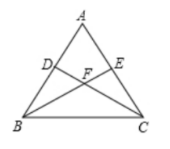(1)判断 $\angle A B E$ 与 $\angle A C D$ 的数量关系, 并说明理由;
(2)求证：过点 $A 、 F$ 的直线垂直平分线段 $B C$.\begin{aligned} & \left\{\begin{array}{l} A B=A C \\ \angle A=\angle A \\ A E=A D \end{array}\right. \\ & \therefore \triangle A B E \cong \triangle A C D, \\ & \therefore \angle A B E=\angle A C D ; \end{aligned}

(2)连接 $A F$.
\begin{aligned} & \because A B=A C, \\ & \therefore \angle A B C=\angle A C B, \\ & \text { 由(1)可知 } \angle A B E=\angle A C D, \\ & \therefore \angle F B C=\angle F C B, \end{aligned}
\begin{aligned} & \therefore F B=F C, \\ & \because A B=A C, \end{aligned}
$\therefore$ 点 $A 、 F$ 均在线段 $B C$ 的垂直平分线上, 即直线 $A F$ 垂直平分线段 $B C$.①点击 首页查看更多试卷和试题 , 点击查看 本题所在试卷